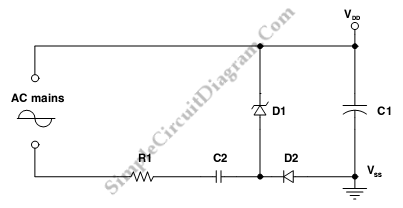# Capacitive AC-DC Adapter

Capacitive power supply is one variation of uninsulated AC to DC adapter. This circuit consist of resistor, capacitors, diode and zener diode. This circuit will allow  the constant voltage to across C1. The input current’s average should be equal to the average value of the output current, to maintain a constant voltage that across C1. Diode D2 is used to half-wave rectify the input current. This is a small size and low cost circuit that easy to implement. However, this circuit has some disadvantages like the standby power consumption is high and has low average output current. Here is the schematic diagram of the circuit:R1 resistor value, The D1 zener voltage, and the input current’s average set the standby power consumption. Besides resistor R1 also used to prevent the overrating of the current protection of the circuit and limit the inrush  current stress at power supply turn on. The Average value of input current is determined by following equation:
Iin(av)=2xfxC2xVmains(peak)=Iout(av)
And the standby power consumption is determined by following equation:
Poff=R1xsquare((Iin(av)x(pi/squareroot(2))))+VzxIin(av)

[Circuit’s schematic diagram source: STMicroelectronics Application Note]